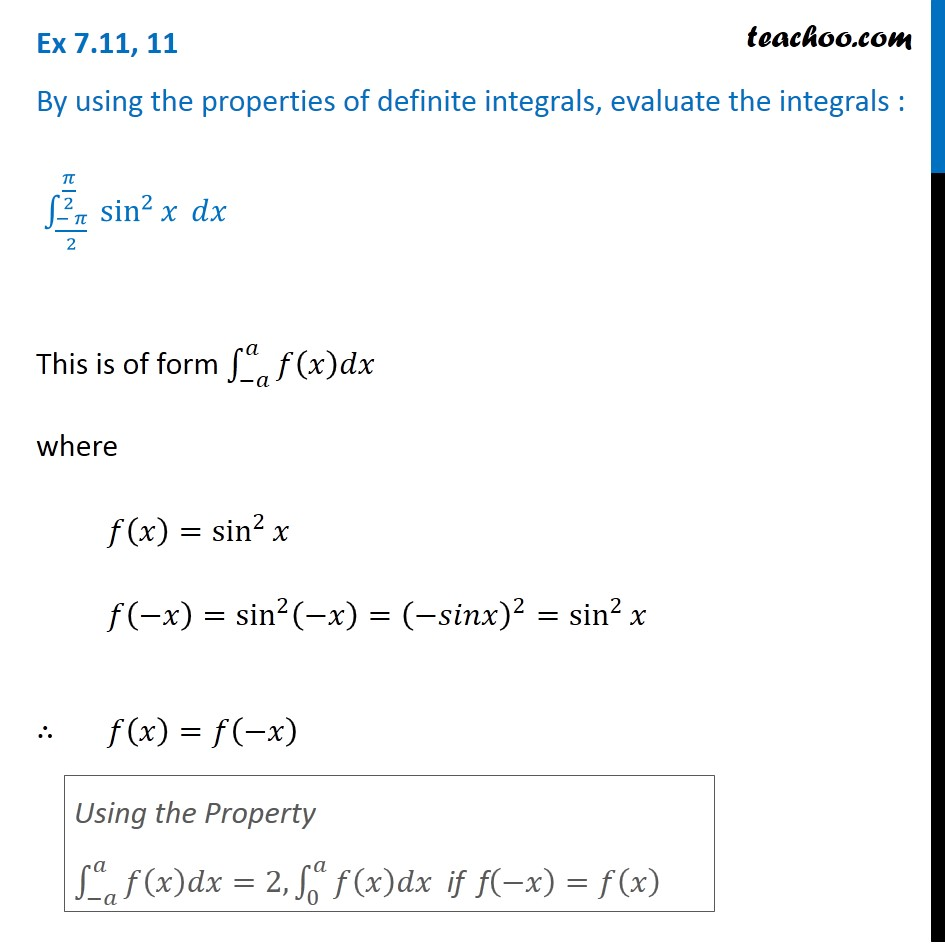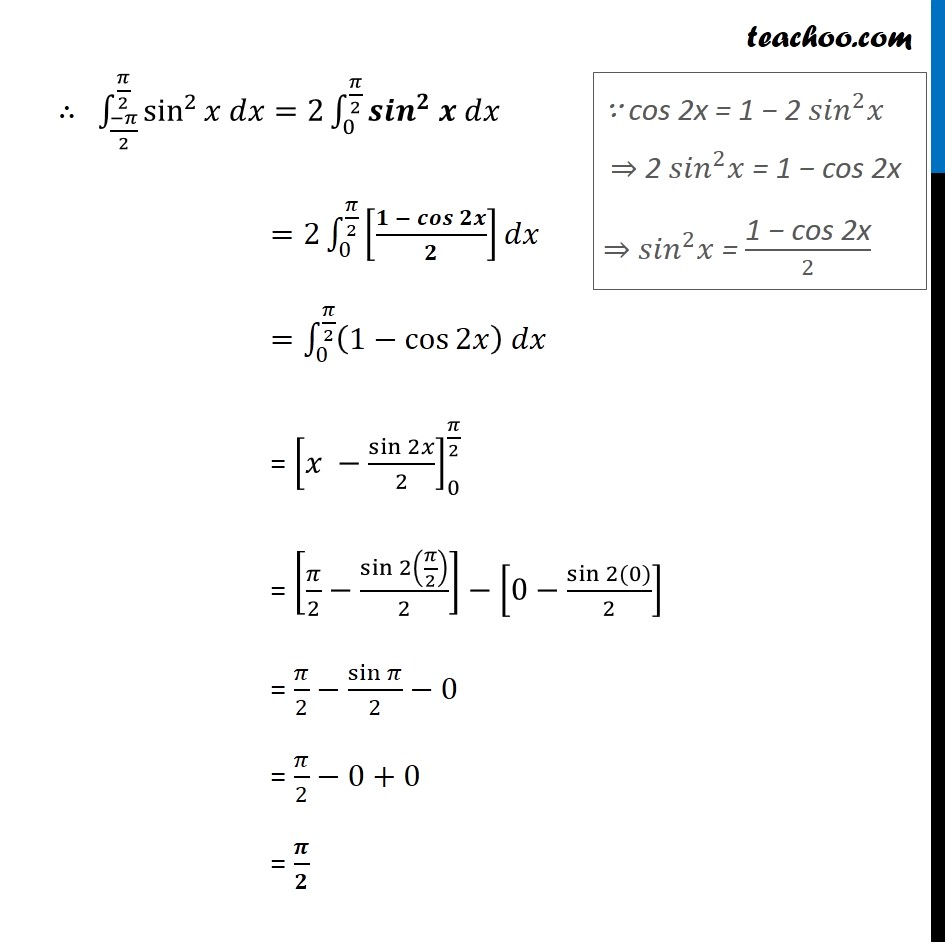1. Chapter 7 Class 12 Integrals (Term 2)
2. Serial order wise
3. Ex 7.11

Transcript

Ex 7.11, 11 By using the properties of definite integrals, evaluate the integrals : ∫_((− 𝜋)/2)^(𝜋/2)▒〖 sin^2〗⁡𝑥 𝑑𝑥 This is of form ∫_(−𝑎)^𝑎▒𝑓(𝑥)𝑑𝑥 where 𝑓(𝑥)=sin^2⁡𝑥 𝑓(−𝑥)=sin^2⁡(−𝑥)=(−𝑠𝑖𝑛𝑥)^2=sin^2⁡𝑥 ∴ 𝑓(𝑥)=𝑓(−𝑥) Using the Property ∫_(−𝑎)^𝑎▒〖𝑓(𝑥)𝑑𝑥=2,〗 ∫_0^𝑎▒〖𝑓(𝑥)𝑑𝑥 〗 if f(−𝑥)=𝑓(𝑥) ∴ ∫_((−𝜋)/2)^(𝜋/2)▒〖sin^2⁡〖𝑥 𝑑𝑥〗=2∫_0^(𝜋/2)▒〖〖𝒔𝒊𝒏〗^𝟐 𝒙 𝑑𝑥〗〗 =2∫_0^(𝜋/2)▒[(𝟏 − 𝒄𝒐𝒔⁡𝟐𝒙)/𝟐]𝑑𝑥 =∫_0^(𝜋/2)▒〖(1−cos⁡2𝑥 ) 𝑑𝑥〗 = [𝑥 −sin⁡2𝑥/2]_0^(𝜋/2) = [𝜋/2−sin⁡2(𝜋/2)/2]− [0−sin⁡〖2(0)〗/2] = 𝜋/2−sin⁡𝜋/2−0 = 𝜋/2−0+0 = 𝝅/𝟐 ∵ cos 2x = 1 − 2 〖𝑠𝑖𝑛〗^2 𝑥 ⇒ 2 〖𝑠𝑖𝑛〗^2 𝑥 = 1 − cos 2x ⇒ 〖𝑠𝑖𝑛〗^2 𝑥 = "1 − cos 2x" /2

Ex 7.11